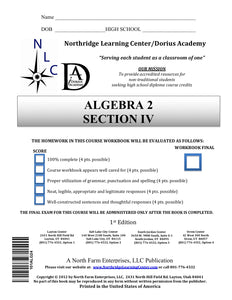# Algebra II, Section IV

Regular price
\$56.50
Regular price
Sale price
\$56.50
Unit price
per
Availability
Sold out

Algebra II 07-02-00-00-020

This course builds on a foundational knowledge of algebra and teaches students how to apply previously learned concepts to solve more complex problems. Students will learn how to solve and graph quadratic, exponential, logarithmic, absolute value, and trigonometric equations. Students will work with equations with both real and imaginary solutions, and will learn to graph equations by using parent functions. By the end of this course, students will be expected to solve basic permutation, combination, and probability problems and solve trigonometry problems using both triangles and the unit circle.